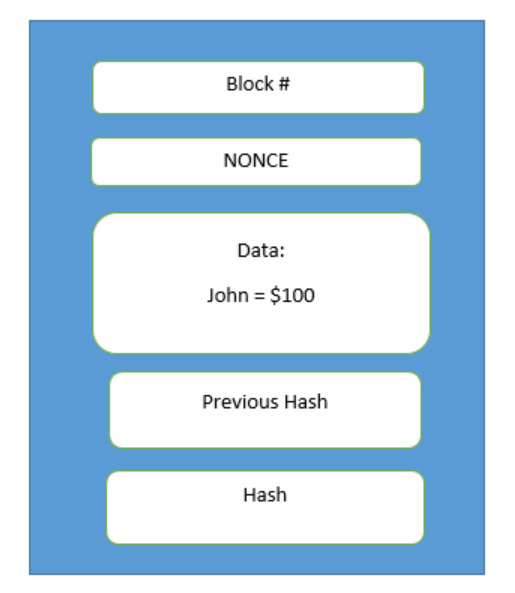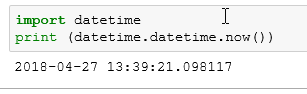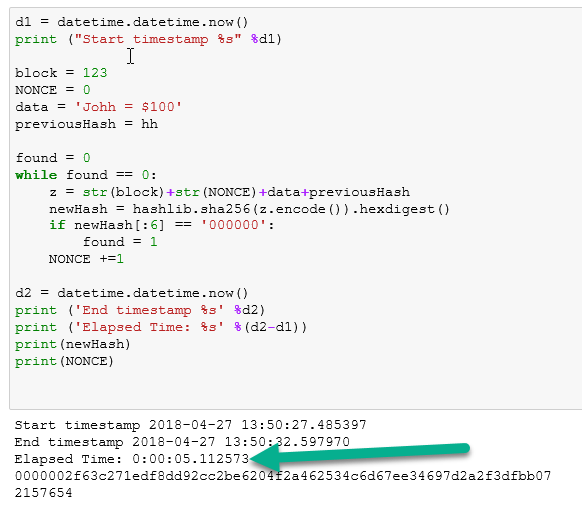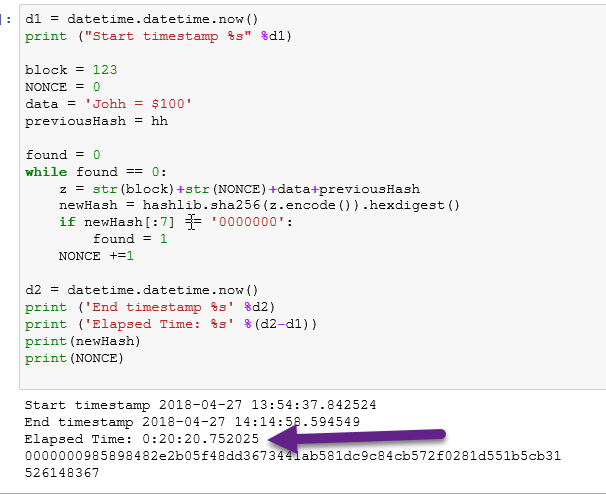# Python: Simulate Blockchain Mining

In my earlier tutorial, I demonstrated how to use the Python library hashlib to create a sha256 hash function. Now, using Python, I am going to demonstrate the principle of blockchain mining. Again using BitCoin as my model, I will be trying to find a nonce value that will result in a hash value below a predetermined target.

We will start by simply enumerating an integer through our sha 256 hash function until we find a hash with 4 leading zeros.

I used a while loop, passing the variable “y” through my hashing function each time the loop runs. I then inspect the first 4 digits [:4] of my hash value. If the first four digits equal 0000 then I exit the loop by setting the found variable to 1

(*note, a hash value is a string – hence the need for quotes around ‘0000’)As you can see in the version above, it took 88445 iterations to find an acceptable hash value

Now, using the basic example of a blockchain I gave in an earlier lesson, let’s simulate mining a blockYou’ll see, I am now combining the block number, nonce, data, and previous hash of my simulated block and passing it through my encryption function.  Just like in BitCoin, the only value I change per iteration is the Nonce. I keep passing my block through the hashing function until I find the Nonce that gives me a hash below the target.Now, let’s lower the target value to 6 leading zeros. This should result in a longer runtime to get your hashTo measure the run time difference, let’s add some time stamps to our codeSo, I am using the timestamp function twice. D1 will be our start time, d2 will be our end time, and I am subtracting d1 from d2 to get our elapsed time. In the example below, my elapsed time was 5 secsNow, let’s bump the target down to 7 leading zeros. Now this brings my elapsed computing time to 20 minutes. That is a considerable commitment of resources. You can see why they call it a “proof of work” now.## 3 thoughts on “Python: Simulate Blockchain Mining”

1.Victor

Hi There,
In your first program (see above) i.e to find a nonce value which results in 4 leading zeros.
In the above example, the nonce value is 88485 and the hash value is 0000a456e…………………
Am I right to say that if I equate y =88485 and run the following:
hh =hashlib.sha256(str(y).encode()).hexdigest()
Will I get the same hash value 0000a456e…………? If not, where have I gone wrong?
2.khanzaman wazir
3.matu inmahda